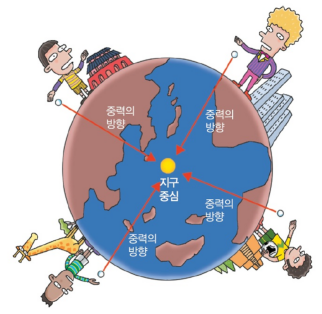# Gravity difference on several planet (with the scale that used on Earth)

Let's measure my weight on several planets with the scale that we used on Earth.

## Newton explaining the gravity mathematically

Every object has a gravitational field around it and attracts surrounding objects. The strength of this gravitational field is proportional to the mass of the object.The concept of gravity is proposed by Newton in the UK for the first time. When an epidemic is prevalent and the school is closed, Newton has discovered gravity law under the apple tree after returning to Britain's home. (It is a story coming, and it is not clear whether it is true.)
Let's officially explain the gravity that Newton found. Newton's gravity can be expressed as follows.$F=G\frac { { m }_{ 1 }{ m }_{ 2 } }{ { r }^{ 2 } }$

Two objects with mass are always attractive. The force is inversely proportional to the square of the distance between the two objects, proportional to the product of the mass of the object. 'F' is short for Force. The unit of force is 'N(Newton).'
The 'G' is a value that is revealed as an accurate experiment result as a proportionality constant.

G ≒ 0.0000000000667 m3/s2kg

≒ 6.67 x 10-11m3/s2kg

In other words, if two objects of 1 kg are about 1 m apart, they are attracting about 6.67 x 10-11N. Since the value of "G" is very tiny, we can see that gravity is a feeble force. Gravity is the weakest force among the four Forces known to date. However, it is also the most powerful force in the universe.

Large objects like the Earth can produce a large gravity field, but small objects around them can not exert a large gravity force to attract each other. Therefore, the most gravity that we can see around us is the gravity of the earth.